## Most Used Formulas (cont.)

 Conductance Admittance In direct current circuits, conductance is expressed  by,where      G = conductance in mhos,                   R = resistance in ohms,   In d-c circuits involving resistances R1, R2, R3, etc.  in parallel,    the total conductance is expressed by             Gtotal = G1 + G2 + G3 ...etc.   and the total current by             Itotal = E Gtotal   and the amount of current in any single  resistor, R2 for example, in a parallel group,   by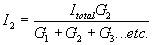R, E and I in Ohm's law formulas for d-c circuits   may be expressed in terms of conductance as follows: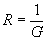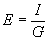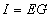where      G  =  conductance in mhos,                   R  =  resistance in ohms,                   E  =  potential in volts,                    I  =  current in amperes, In an alternating current circuit, the admittance of a series  circuit is expressed by,Admittance is also expressed as the reciprocal of  impedance, or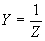where      Y = admittance in mhos,                  R = resistance in ohms,                  X = reactance in ohms,                  Z = impedance in ohms, R and X in Terms of G and B Resistance and reactance may be expressed in terms  of conductance and susceptance as follows: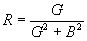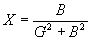G, B, Y and Z in Parallel Circuits Susceptance In any given a-c circuit containing a number of smaller   parallel circuits only,      the effective conductance GT is expressed by                 GT = G1 + G2 + G3...etc.      and the effective susceptance BT by                 BT = B1 + B2 + B3...etc.      and the effective admittance YT by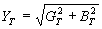and the effective impedance ZT by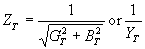where       R  =  resistance in ohms,                   X  =  reactance (capacitive or induc-                           tive) in ohms,                   G  =  conductance in mhos,                    B  =  susceptance in mhos,                    Y  =  admittance in mhos,                    Z  =  impedance in ohms, In an alternating current circuit, the susceptance of a   series circuit is expressed by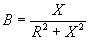or, when the resistance is 0, susceptance becomes   the reciprocal of reactance, orwhere    B  =  susceptance in mhos,                R  =  resistance in ohms,                X  =  reactance in ohms,